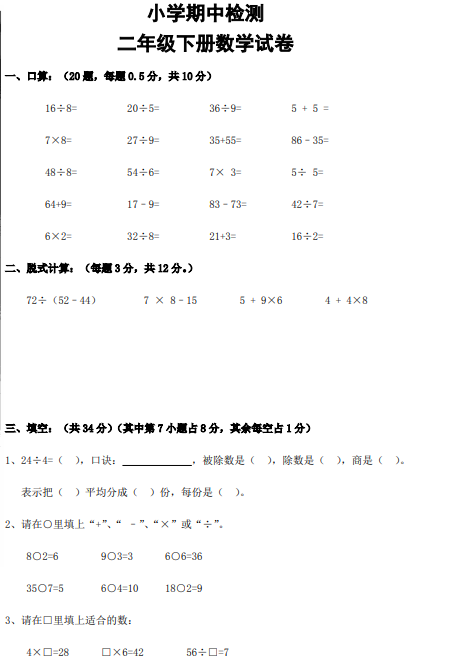16÷8= 20÷5= 36÷9= 5 + 5 =
7×8= 27÷9= 35+55= 86–35=
48÷8= 54÷6= 7× 3= 5÷ 5=
64+9= 17–9= 83–73= 42÷7=

【PDF文档4页】新人教版小学二年级下学期数学期中试卷 (第四份)A4电子版资料_可直接打印_会员免费下载##### 评论信息